# R Notes¶

## Installation¶

### Install from source on Rocky¶

Following the instruction in RStudio Documentation: Install R from Source

export R_VERSION=X.X.X
curl -O https://cran.rstudio.com/src/base/R-4/R-${R_VERSION}.tar.gz tar -xzvf R-${R_VERSION}.tar.gz
cd R-${R_VERSION}  Build with ./configure \ --prefix=/opt/R/${R_VERSION} \
--enable-memory-profiling \
--enable-R-shlib \
--with-blas \
--with-lapack

make
sudo make install


then manage the version with module.

2022-08-24 10:32:20

On T460P, change the prefix as follows

./configure \
--prefix=/home/weiya/Programs/R/${R_VERSION} \ --enable-memory-profiling \ --enable-R-shlib \ --with-blas \ --with-lapack  #### installation of module¶ Follow the official instructioncurl -LJO https://github.com/cea-hpc/modules/releases/download/v5.1.1/modules-5.1.1.tar.gz tar xvf modules-5.1.1.tar.gz cd modules-5.1.1/ sudo apt-get install tcl-dev make # sudo make install make install  Different from other software, module load command cannot be found, we need to configure as follows, $ sudo ln -s $PWD/init/profile.sh /etc/profile.d/modules.sh$ sudo ln -s $PWD/init/profile.csh /etc/profile.d/modules.csh  So the above make install indeed does not need sudo. Strangely, it still cannot find module, (require reboot?), so simply put source /etc/profile.d/modules.sh into ~/.bashrc ### Install 4.1.0 from source¶ it throws when ./configure, configure: error: libcurl >= 7.28.0 library and headers are required with support for https  try to install libcurl4-openssl-dev, suggested in Installing R on Linux: configure: error: libcurl >= 7.28.0 library and headers are required with support for https. Be careful when installing the package, and to avoid the uninstallation in the next section. !!! “2022-08-24 10:35:01” Run sudo apt-get install libcurl4-openssl-dev, and monitor the message, no packages are needed to be removed. ### Install Latest R3.6¶ Accidentally, I uninstall my R3.6 when installing libcurl4, the apt-log shows that Start-Date: 2021-05-01 10:40:15 Commandline: apt install libcurl4 Requested-By: weiya (1000) Install: libcurl4:amd64 (7.58.0-2ubuntu3.13) Remove: r-cran-foreign:amd64 (0.8.75-1xenial0), r-cran-mgcv:amd64 (1.8-31-1cran1xenial0), libcurl3:amd64 (7.58.0-2ubuntu2), r-base-html:amd64 (3.6.3-1xenial), r-cran-class:amd64 (7.3-16-1xenial0), r-cran-boot:amd64 (1.3-23-2xenial0), r-cran-cluster:amd64 (2.1.0-2xenial0), r-cran-rpart:amd64 (4.1-15-2xenial0), r-cran-kernsmooth:amd64 (2.23-16-1+1xenial0), r-cran-matrix:amd64 (1.2-18-1xenial0), r-cran-spatial:amd64 (7.3-11-2xenial0), r-base-core:amd64 (3.6.3-1xenial), r-cran-codetools:amd64 (0.2-16-1xenial0), r-base:amd64 (3.6.3-1xenial), r-recommended:amd64 (3.6.3-1xenial), r-cran-nlme:amd64 (3.1.144-1xenial0), r-cran-lattice:amd64 (0.20-41-1cran1xenial0), r-cran-nnet:amd64 (7.3-13-1xenial0), r-cran-mass:amd64 (7.3-51.5-1xenial0), r-cran-survival:amd64 (3.1-11-1cran1xenial0) End-Date: 2021-05-01 10:40:29  So I need to reinstall it. Following the instruction on CRAN 1. add deb https://cloud.r-project.org/bin/linux/ubuntu bionic-cran35/ to /etc/apt/sources.list, actually I found a xenial version, which means that I had followed the procedure on Ubuntu 16.04. Currently I am on Ubuntu 18.04, so I use a bionic version. 2. run sudo apt-get update, but it throws an error, W: GPG error: https://cloud.r-project.org/bin/linux/ubuntu bionic-cran35/ InRelease: The following signatures were invalid: EXPKEYSIG 51716619E084DAB9 Michael Rutter marutter@gmail.com E: The repository ‘https://cloud.r-project.org/bin/linux/ubuntu bionic-cran35/ InRelease’ is not signed. N: Updating from such a repository can’t be done securely, and is therefore disabled by default. N: See apt-secure(8) manpage for repository creation and user configuration details. The error has been reported in Linux#GPG error, and the solution is sudo apt-key adv --keyserver keyserver.ubuntu.com --recv-keys 51716619E084DAB9  1. run sudo apt-get install r-base. ## Multiple Versions¶ ### prefix + module (optionally)¶ specify --prefix when ./configure, e.g. Installing multiple versions of R on Linux – RStudio Support ### Conda¶ Using R language with Anaconda • 单独安装 rstudio conda install -c r rstudio (waste memory!!) • 共用 Rstudio，但是切换环境时找不到 shared library，而原生 R session 完全没问题。目前解决方案，命令行调用 rstudio 之前添加环境变量 conda activate R4.1.0 export LD_LIBRARY_PATH=/media/weiya/PSSD/Programs/anaconda3/envs/R4.1.0/lib:$LD_LIBRARY_PATH
rstudio


## sys.nframe()¶

Here is an equivalent way for pythonic if __name__ == "__main__"

# runs only when script is run by itself
if (sys.nframe() == 0){
# ... do main stuff
}


Example

My Repos

## Common Tips¶

• 删除当前工作区所有变量: rm(list = ls(all = TRUE))
• RStudio shows all shortcuts: Alt-Shift-K.

## sort(), rank(), order()¶

• sort(x): sort x increasingly (by default)
• rank(x): the ranks of each element
• order(x): the permutation for sort(x)
> x = c(97, 93, 85, 74, 32, 100, 99, 67)
> sort(x)
  32  67  74  85  93  97  99 100
> order(x)
 5 8 4 3 2 1 7 6
> rank(x)
 6 5 4 3 1 8 7 2


and they satisfy (assuming no ties and in increasing order)

\begin{align*} \mathrm{x[order(x)]} & = \mathrm{sort(x)}\\ \mathrm{rank(x)} &= \mathrm{order(order(x))}\\ \mathrm{sort(x)[rank(x)]} &= \mathrm{x} \end{align*}
> x[order(x)]
  32  67  74  85  93  97  99 100
> order(order(x))
 6 5 4 3 1 8 7 2


In particular, if x = 1:n, then x = order(x) = sort(x), and hence

\mathrm{x[x] = x}

Python uses np.argsort to return the index of the sorted array, such as

>>> a = np.array([97, 93, 85, 74, 32, 100, 99, 67])
>>> sorted(a)
[32, 67, 74, 85, 93, 97, 99, 100]
>>> idx = np.argsort(a)
[32, 67, 74, 85, 93, 97, 99, 100]
>>> a[idx]
array([ 32,  67,  74,  85,  93,  97,  99, 100])


but keep in mind that the index starts from 0 instead of 1. Here is another way in Python | Returning index of a sorted list (TODO).

In julia, we use sort and sortperm.

## Run from Command Line¶

• run R -q -e "1+1" in the command line
• run Rscript test.R in the command line
• put #!/usr/bin/env Rscript on the first line of test.R and allow it executable via chmod +x test.R

To pass arguments, we can use

args = commandArgs(trailingOnly=TRUE)


to extract the arguments.

Test with the following scripts

args = commandArgs(trailingOnly=TRUE)
print(length(args))
cat(args)

args = commandArgs(trailingOnly=FALSE)
print(length(args))
cat(args)


the results are

$Rscript test1.R xxx yyy  2 xxx yyy$ Rscript test2.R xxx yyy
 7
/usr/lib/R/bin/exec/R --slave --no-restore --file=test2.R --args xxx yyy


which shows that trailingOnly=TRUE is necessary and it only takes the arguments after --args.

## Round numbers¶

I want to approximate a numerical value with 3 significant digits, but the trailing zeros are always be dropped, then I tried several methods.

First of all, define

p <- function(x) { cat(deparse(substitute(x)), "=", x, "\n") }


for convenient and clear comparisons, which takes the advantage of R’s Lazy Evaluation.

x = 0.04898246 # want 0.0490
p(signif(x, digits=3))
p(prettyNum(x))
p(prettyNum(x, digits=3))
p(formatC(x, drop0trailing = F))
p(formatC(x, drop0trailing = T))
p(format(signif(x, digits=3), nsmall = 3))
p(format(signif(x, digits=3), nsmall = 4))
p(format(signif(x, digits=3), nsmall = 3 + floor(-log10(x))))
# signif(x, digits = 3) = 0.049
# prettyNum(x) = 0.04898246
# prettyNum(x, digits = 3) = 0.049
# formatC(x, drop0trailing = F) = 0.04898
# formatC(x, drop0trailing = T) = 0.04898
# format(signif(x, digits = 3), nsmall = 3) = 0.049
# format(signif(x, digits = 3), nsmall = 4) = 0.0490
# format(signif(x, digits = 3), nsmall = 3 + floor(-log10(x))) = 0.0490


where floor(-log10(x)) returns the number of zeros between the decimal symbol . and the first nonzero value (assume x < 1).

With scientific = TRUE option, nsmall would fail,

p(format(signif(x, digits=3), scientific = T, nsmall = 3 + floor(-log10(x))))
# format(signif(x, digits = 3), scientific = T, nsmall = 3 + floor(-log10(x))) = 4.9e-02


as the manual ?format said,

the minimum number of digits to the right of the decimal point in formatting real/complex numbers in non-scientific formats. Allowed values are 0 <= nsmall <= 20.

For a larger value, such as 37289.75, the scientific form might be necessary,

y = 37289.75
p(format(signif(y, digits=3), nsmall= max(0, 3 + floor(-log10(y))) ) )
p(format(signif(y, digits=3), scientific = T))
# format(signif(y, digits = 3), nsmall = max(0, 3 + floor(-log10(y)))) = 37300
# format(signif(y, digits = 3), scientific = T) = 3.73e+04


Finally, I found that sprintf("%.2e", x) would be helpful, and actually the approximation has been consider, instead of just truncating.

p(sprintf("%.2e, %.1e", x, y))
#sprintf("%.2e, %.1e", x, y) = 4.90e-02, 3.7e+04


## 序列减去常数¶

for (i in c(1:n-1))
print(i)
##0
##1
##2
for (i in c(1:(n-1)))
print(i)
##1
##2


## Rstudio 清空历史图象¶

Error in plot.new() : figure margins too large in R## window 安装包¶

## set a CRAN mirror
local({r <- getOption("repos")
r["CRAN"] <- "http://mirrors.ustc.edu.cn/CRAN/"
options(repos=r)})


## Interpreting Residual and Null Deviance in GLM R¶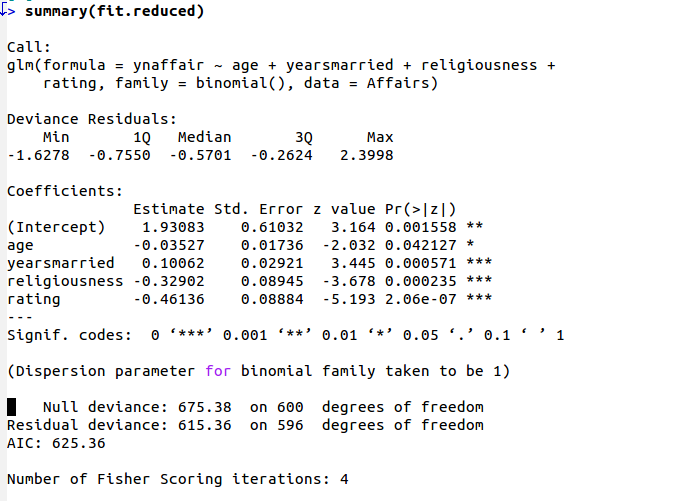## 缺少libRblas.so和libRlapack.so的解决办法¶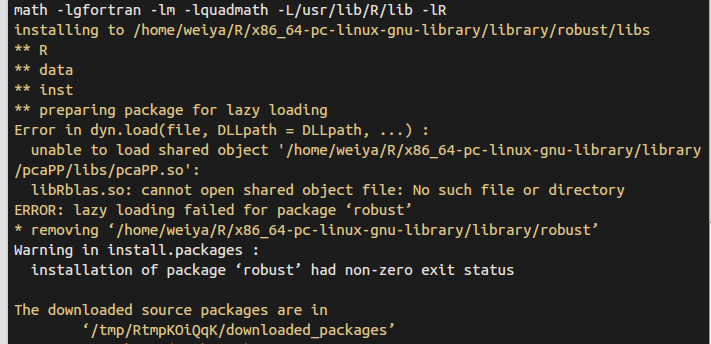cd /usr/lib
ln -s libblas.so libRblas.so
ln -s /usr/lib/R/module/lapack.so libRlapack.so


## Rcpp¶

Info

Another repo for learning Rcpp: RcppNotes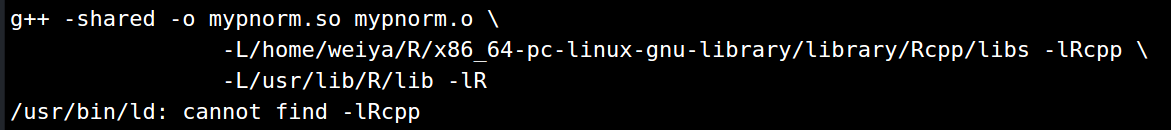cd /usr/local/lib
##cd /usr/lib
ln -s /home/weiya/R/x86_64-pc-linux-gnu-library/library/Rcpp/libs/Rcpp.so libRcpp.so


### function ‘dataptr’ not provided by package ‘Rcpp’¶

dyn.load()


library(Rcpp)
## 或require(Rcpp)


check locale

## par cheatsheet¶

r-graphical-parameters-cheatsheet

## Mathematical Annotation in R plot¶

plot(..., main = expression(paste("...", mu)))


## remove outliers from the boxplot¶

How to remove outliers from a dataset

## rmarkdown转化中文字符为PDF的设置¶

---
title: "test"
author: "weiya"
output:
pdf_document:
latex_engine: xelatex
includes:
---


## rmarkown compiler does not show captions for two consecutive figures¶

add at least two spacing newline.

Some figure captions from RMarkdown not showing

## 在grid排列图¶

Arranging plots in a grid

## semi-transparency is not supported on this device¶

semi-transparency is not supported on this device

## MC, MCMC, Gibbs采样 原理¶

MC, MCMC, Gibbs采样 原理&实现（in R）

DP: Collapsed Gibbs Sampling

Metropolis Hasting算法

## Running R in batch mode on Linux¶

Running R in batch mode on Linux

## “Kernel density estimation” is a convolution of what?¶

“Kernel density estimation” is a convolution of what?

## unable to start rstudio in centos getting error “unable to connect to service”¶

unable to start rstudio in centos getting error “unable to connect to service”

## 发布R包¶

Releasing a package

## Presentations with Slidy¶

Presentations with Slidy

## Estimation of the expected prediction error¶

Estimation of the expected prediction error

## ROCR包中prediction函数¶

prediction定义如下

prediction(predictions, labels, label.ordering = NULL)


## generate some data with a non-linar class boundary
set.seed(123)
x = matrix(rnorm(200*2), ncol = 2)
x[1:100, ] = x[1:100, ] + 2
x[101:150, ] = x[101:150, ] - 2
y = c(rep(1, 150), rep(2, 50))
dat = data.frame(x = x, y = as.factor(y))
plot(x, col = y)

## randomly split into training and testing groups
train = sample(200, 100)

## training data using radial kernel
svmfit = svm(y~., data = dat[train, ], kernel = "radial", cost = 1)
plot(svmfit, dat[train, ])

## cross-validation
set.seed(123)
tune.out = tune(svm, y~., data = dat[train, ], kernel = "radial",
ranges = list(cost = c(0.1, 1, 10, 100, 1000),
gamma = c(0.5, 1, 2, 3, 4)))
summary(tune.out)

## prediction
table(true = dat[-train, "y"], pred = predict(tune.out$best.model, newdata = dat[-train, ])) ## ROC curves library(ROCR) rocplot = function ( pred , truth , ...) { predob = prediction ( pred, truth , label.ordering = c("2", "1")) perf = performance ( predob , "tpr" , "fpr") plot ( perf,...) } svmfit.opt = svm(y~., data = dat[train, ], kernel = "radial", gamma = 3, cost = 10, decision.values = T) fitted = attributes(predict(svmfit.opt, dat[train, ], decision.values = T))$decision.values

rocplot ( fitted , dat [ train ,"y"] , main ="Training Data")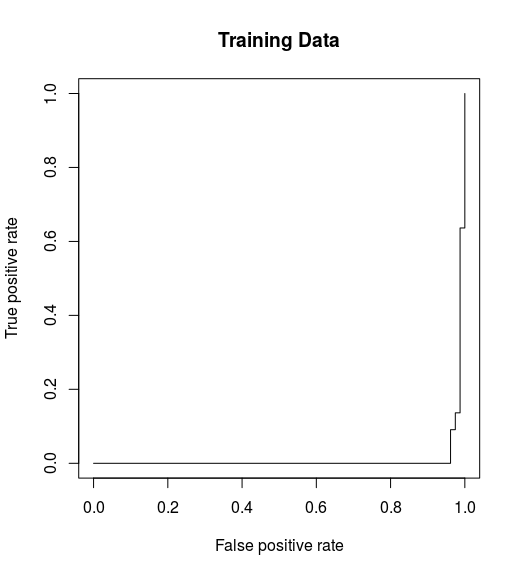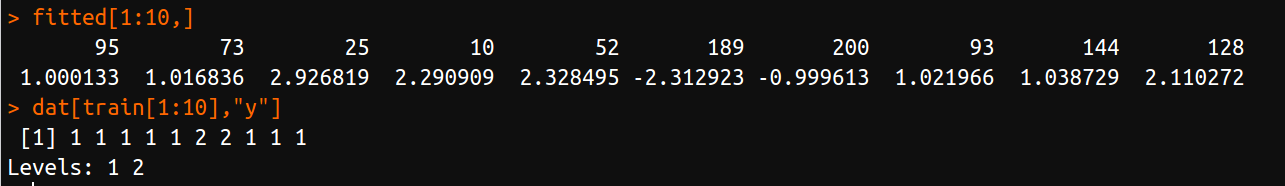## 神奇的[¶

A = array(sample(0:255, 100*100*3, replace = T), dim = c(100,100,3))
B = array(sample(1:100, 2*5), dim = c(2,5))
apply(A, 3, [, t(B))


## lm() 中有无 I() 的差异¶

lm(Y ~ X + X^2)


lm(Y ~ X + I(X^2))


## custom print¶

class(obj) = "example"
print.example <- function(x)
{

}


## write lines to file¶

fileConn<-file("output.txt")
writeLines(c("Hello","World"), fileConn)
close(fileConn)


## specify CRAN mirror in install.package¶

r <- getOption("repos")
r["CRAN"] <- "https://cran.r-project.org"
# r["CRAN"] <- "r["CRAN"] <- "https://mirrors.ustc.edu.cn/CRAN/"" ## for mainland China
options(repos=r)


we also can wrap it with local({...}) and save in ~/.Rprofile.

Refer to How to select a CRAN mirror in R

For temporary use, use repos argument in install.packages, such as

install.packages('RMySQL', repos='http://cran.us.r-project.org')


refer to How to select a CRAN mirror in R

## R 符号运算¶

NormDensity <- expression(1 / sqrt(2 * pi) * exp(-x^2 / 2))
D(NormDensity, "x")
DD <- function(expr, name, order = 1) {
if (order < 1)
stop("'order' must be >= 1")
if (order == 1)
D(expr, name) else DD(D(expr, name), name, order - 1)
}
DD(NormDensity, "x", 3)

DFun <- deriv(NormDensity, "x", function.arg = TRUE)
DFun(1)


## Mistake with colon operator¶

vec <- c()
for (i in 1:length(vec)) print(vec[i])


would print two NULL because 1:length(vec) would be c(1,0). A method to avoid this

for (i in seq_along(vec)) print(vec[i])


## error in install gRbase¶

environment: Ubuntu 16.04 (gcc 5.4.0)

g++: error: unrecognized comma line option ‘-fno-plt’


the reason should be that the current gcc is too old.

In conda env R:

1. install latest gcc v7.3.0, but it still does not work
2. Sys.getenv() indeed switch to the latest gcc
3. remove ~/.R/Makevars, which would force the gcc to be the gcc declared in that file.
4. then it works well.

refer to

R Packages Fail to Compile with gcc

Note that some packages cannot be installed via CRAN, and you can check bioconductor.

if (!requireNamespace("BiocManager", quietly = TRUE))
install.packages("BiocManager")

BiocManager::install("graph")


## protection stack overflow¶

use

R --max-ppsize 500000


or for rstudio

rstudio --max-ppsize 500000


## not found libhdf5.a¶

check if compiling under anaconda environment, if so, exit and retry.

## install.packages returns failed to create lock directory¶

in bash

R CMD INSTALL --no-lock <pkg>


or in R session

install.packages("Rcpp", dependencies=TRUE, INSTALL_opts = c('--no-lock'))


## plot.new() : figure margins too large¶

Rstudio 中对于太大的图片有可能报错，比如当我试图以 par(mfrow=c(4,1)) 画四个 matplot，于是报错。这时候，可以直接在 R session 里面绘制。

## S3 method¶

j = list(name = "Joe", salary = 5500, union = T)
class(j) = "employee"

print.employee <- function(wrkr){
cat(wrkr$name, "\n") cat("salary", wrkr$salary, "\n")
cat("union member", wrkr$union, "\n") } summary.employee <- function(wrkr){ cat(wrkr$name, "\n")
cat("salary", wrkr$salary, "\n") cat("union member", wrkr$union, "\n")
}


## Parallel Computing¶

• parallel: makeCluster and stopCluster
• doParallel: registerDoParallel
• foreach: %dopar%

### example¶

adapt from my project

cl <- makeCluster(ncl)
registerDoParallel(cl)

res = foreach(j=1:Nnset, .combine = 'c', .export = c('calc_lda_BIC'), .packages = 'nnet') %dopar%
{
jj = not_set[j];
new_set   = sort(c(jj, cur_set));
new_score = calc_lda_BIC(xx, yy, new_set, D, K, debug, gam=gam);
new_score
}
stopCluster(cl)


## using R in JupyterLab¶

install.packages('IRkernel')
#IRkernel::installspec()
IRkernel::installspec(name="3.6.0", displayname = "R 3.6.0")


refer to IRkernel

and try to use jupyterlab, which seems much powerful than jupyter.

But note that -X option is needed before creating a tmux session for running jupyter, otherwise the kernel keeps dead in the browser and check the monitor message from jupyter, which throws,

Error in .External2(C_X11, paste0("png::", filename), g$width, g$height,  :
unable to start device PNG
Calls: <Anonymous> ... evaluate -> dev.new -> do.call -> <Anonymous> -> ok_device
In addition: Warning message:
In ok_device(filename, ...) : unable to open connection to X11 display ''
Execution halted


although I am just trying to calculate 1+1, nothing related to X11.

The error would appear again if the -X ssh session has been stopped. Then I found such issue has been reported in https://github.com/IRkernel/IRkernel/issues/388, and there might be solution by https://github.com/IRkernel/IRkernel/issues/388#issuecomment-237080809, but the working R version is built from source by myself, and Cairo is not supported. When I am trying to install the package, it throws that

configure: error: Cannot find cairo.h! Please install cairo (http://www.cairographics.org/) and/or set CAIRO_CFLAGS/LIBS correspondingly.
ERROR: configuration failed for package ‘Cairo’


Even I retried after specifying

export CAIRO_LIBS=/home/project09/miniconda3/envs/r3.6.3/lib
export CAIRO_CFLAGS=/home/project09/miniconda3/envs/r3.6.3/include


by linking it to other R version with Cairo support, similar as in https://stat.ethz.ch/pipermail/r-help/2014-April/374096.html, but it does not work.

Then I change to a different R version with cairo support, and it can work without -X option. And the setting in .Rprofile can also been removed (or actually the default is exactly the same).

Try to install the shortcuts as recommended, but it throws,

$jupyter labextension install @techrah/text-shortcuts An error occured. ValueError: Please install Node.js and npm before continuing installation. You may be able to install Node.js from your package manager, from conda, or directly from the Node.js website (https://nodejs.org). See the log file for details: /tmp/jupyterlab-debug-7mmu8jy1.log  then conda install -c conda-forge nodejs  as suggested in Extensions However the default version is outdated. libgcc-7.2.0 | 304 KB | ############################################################################################################################################# | 100% nodejs-6.13.1 | 11.9 MB | ############################################################################################################################################# | 100% Preparing transaction: done Verifying transaction: done Executing transaction: done (base) [project09@stapc390 ~]$ jupyter labextension install @techrah/text-shortcuts
An error occured.
ValueError: Please install nodejs >=10.0.0 before continuing. nodejs may be installed using conda or directly from the nodejs website.
See the log file for details:  /tmp/jupyterlab-debug-lhf6bjwm.log


The solution is quite easy, just use the default conda channel,

conda install nodejs


which will install nodejs-10.13.0.

> mtcars$transmission = factor(mtcars$am, levels = c(0, 1), labels = c("Automatic", "Manual"))
> str(mtcars)
'data.frame':   32 obs. of  12 variables:
...
$am : num 1 1 1 0 0 0 0 0 0 0 ...$ gear        : num  4 4 4 3 3 3 3 4 4 4 ...
$carb : num 4 4 1 1 2 1 4 2 2 4 ...$ transmission: Factor w/ 2 levels "Automatic","Manual": 2 2 2 1 1 1 1 1 1 1 ...
> data("mtcars")
> str(mtcars)
'data.frame':   32 obs. of  11 variables:
...
$am : num 1 1 1 0 0 0 0 0 0 0 ...$ gear: num  4 4 4 3 3 3 3 4 4 4 ...
$carb: num 4 4 1 1 2 1 4 2 2 4 ...  ## scale or not¶ 下面两个结果是一样的。 m = matrix(rnorm(4*6, 2), ncol = 4) cov(m) sm = scale(m, scale = FALSE) # only center, no scale. t(sm) %*% sm/ (6-1)  ## Check Memory Usage¶ memuse = function () { strtoi(system(paste("ps -p", Sys.getpid(), "-o rss="), intern = T)) / 1024 }  ## Stats Functions¶ • stats::ecdf: empirical cumulative distribution function ## Update Library Path¶ Info Post: 2022-08-30 12:00:39 +0800 The disk is nearly occupied, and I found that $ du -sh *
2.6G    R


which stores the installed packages for R3.6. I want to move it to ~/Programs/R, which is on a portable SSD, so I need to update .libPaths().

The personal path is defined in /usr/lib/R/etc/Renviron

R_LIBS_USER=${R_LIBS_USER-'~/R/x86_64-pc-linux-gnu-library/3.6'}  Replace it with R_LIBS_USER=${R_LIBS_USER-'~/Programs/R/x86_64-pc-linux-gnu-library/3.6'}


Tip

Start from R4.0.0, the path string can be constructed via r(...) without escaping /.

> r"(~/R/x86_64-pc-linux-gnu-library/3.6)"
 "~/R/x86_64-pc-linux-gnu-library/3.6"


## String¶

• repeat string: strrep('str', 2), paste(rep('str', 2), collapse='')